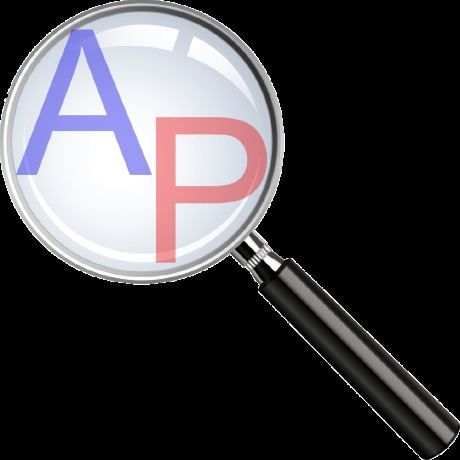# APMonitor

Updated on
Covid-19
 Developer(s)  APMonitorType  Technical computingWebsite  APMonitor product page Operating system  Cross-platformLicense  Proprietary, BSDStable release  v0.7.1 / August 1, 2016 (2016-08-01)

Advanced process monitor (APMonitor), is a modeling language for differential algebraic (DAE) equations. It is a free web-service for solving representations of physical systems in the form of implicit DAE models. APMonitor is suited for large-scale problems and allows solutions of linear programming, integer programming, nonlinear programming, nonlinear mixed integer programming, dynamic simulation, moving horizon estimation, and nonlinear model predictive control. APMonitor does not solve the problems directly, but calls nonlinear programming solvers such as APOPT, BPOPT, IPOPT, MINOS, and SNOPT. The APMonitor API provides exact first and second derivatives of continuous functions to the solvers through automatic differentiation and in sparse matrix form.

## Programming Language Integration

Julia, MATLAB, Python are mathematical programming languages that have APMonitor integration through web-service APIs. The interfaces are built-in optimization toolboxes or modules to both load and process solutions of optimization problems. APMonitor is an object-oriented modeling language and optimization suite that relies on programming languages to load, run, and retrieve solutions. APMonitor models and data are compiled at run-time and translated into objects that are solved by an optimization engine such as APOPT or IPOPT. The optimization engine is not specified by APMonitor, allowing several different optimization engines to be switched out. The simulation or optimization mode is also configurable to reconfigure the model for dynamic simulation, nonlinear model predictive control, moving horizon estimation or general problems in mathematical optimization.

As a first step in solving the problem, a mathematical model is expressed in terms of variables and equations such as the Hock & Schittkowski Benchmark Problem #71 used to test the performance of nonlinear programming solvers. This particular optimization problem has an objective function min x R x 1 x 4 ( x 1 + x 2 + x 3 ) + x 3 and subject to the inequality constraint x 1 x 2 x 3 x 4 25 and equality constraint x 1 2 + x 2 2 + x 3 2 + x 4 2 = 40 . The four variables must be between a lower bound of 1 and an upper bound of 5. The initial guess values are x 1 = 1 , x 2 = 5 , x 3 = 5 , x 4 = 1 . This mathematical model is translated into the APMonitor modeling language in the following text file.

The problem is then solved in Python by first installing the APMonitor package with pip install APMonitor or from the following Python code.

Installing a Python is only required once for any module. Once the APMonitor package is installed, it is imported and the apm_solve function solves the optimization problem. The solution is returned to the programming language for further processing and analysis.

Similar interfaces are available for MATLAB and Julia with minor differences from the above syntax. Extending the capability of a modeling language is important because significant pre- or post-processing of data or solutions is often required when solving complex optimization, dynamic simulation, estimation, or control problems.

## High Index DAEs

The highest order of a derivative that is necessary to return a DAE to ODE form is called the differentiation index. A standard way for dealing with high-index DAEs is to differentiate the equations to put them in index-1 DAE or ODE form (see Pantelides algorithm). However, this approach can cause a number of undesirable numerical issues such as instability. While the syntax is similar to other modeling languages such as gProms, APMonitor solves DAEs of any index without rearrangement or differentiation. As an example, an index-3 DAE is shown below for the pendulum motion equations and lower index rearrangements can return this system of equations to ODE form (see Index 0 to 3 Pendulum example).

## Applications in APMonitor Modeling Language

Many physical systems are naturally expressed by differential algebraic equation. Some of these include:

• cell cultures
• chemical reactors
• cogeneration (power and heat)
• distillation columns
• drilling automation
• friction stir welding
• hydrate formation in deep-sea pipelines
• oscillators
• severe slugging control
• solar thermal energy production
• solid oxide fuel cells
• space shuttle launch simulation
• Unmanned Aerial Vehicles (UAVs)
• Models for a direct current (DC) motor and blood glucose response of an insulin dependent patient are listed below. They are representative of differential and algebraic equations encountered in many branches of science and engineering.

## References

Similar Topics
Rehguzar
Malek Aït Alia
Parthiv Shah
Topics